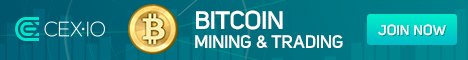# Complex Number Calculator

A complex number is a number has the form a real number plus an imaginary number (a + bi). There are 3 methods of calculating a Complex number Multiplication, Division and Square Root. Back to business tools.

## Complex Number Solver

 a: bi: a: bi:
 Multiplication : [ (a+bi) x (a+bi) ] Division: [ (a+bi) / (a+bi) ]
 Square root: r1 = x+yi Square root: r2 = -x-yi

## Do you know the meaning of any of the following?click here to learn how to turn on javascript

Some of these programs, gadgets and widgets are provided by other websites, we can not be held responsible for the data it provides and how it preforms. If anything seems wrong please let us know straight away and we will either correct, improve or remove.